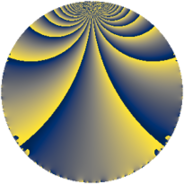# Properties

 Label 3744.2.a.baLevel $3744$ Weight $2$ Character orbit 3744.a Self dual yes Analytic conductor $29.896$ Analytic rank $0$ Dimension $3$ CM no Inner twists $1$

# Learn more

## Newspace parameters

 Level: $$N$$ $$=$$ $$3744 = 2^{5} \cdot 3^{2} \cdot 13$$ Weight: $$k$$ $$=$$ $$2$$ Character orbit: $$[\chi]$$ $$=$$ 3744.a (trivial)

## Newform invariants

 Self dual: yes Analytic conductor: $$29.8959905168$$ Analytic rank: $$0$$ Dimension: $$3$$ Coefficient field: 3.3.148.1 Defining polynomial: $$x^{3} - x^{2} - 3 x + 1$$ Coefficient ring: $$\Z[a_1, \ldots, a_{7}]$$ Coefficient ring index: $$2^{2}$$ Twist minimal: no (minimal twist has level 1248) Fricke sign: $$-1$$ Sato-Tate group: $\mathrm{SU}(2)$

## $q$-expansion

Coefficients of the $$q$$-expansion are expressed in terms of a basis $$1,\beta_1,\beta_2$$ for the coefficient ring described below. We also show the integral $$q$$-expansion of the trace form.

 $$f(q)$$ $$=$$ $$q + ( -1 - \beta_{1} ) q^{5} + \beta_{2} q^{7} +O(q^{10})$$ $$q + ( -1 - \beta_{1} ) q^{5} + \beta_{2} q^{7} + ( 1 - \beta_{1} - \beta_{2} ) q^{11} + q^{13} -2 q^{17} -\beta_{2} q^{19} + 2 \beta_{2} q^{23} + ( 5 + 2 \beta_{1} + 2 \beta_{2} ) q^{25} -2 q^{29} + ( 2 - 2 \beta_{1} - \beta_{2} ) q^{31} + ( 2 - 2 \beta_{1} ) q^{35} -2 \beta_{1} q^{37} + ( -3 + \beta_{1} ) q^{41} + ( -2 - 2 \beta_{1} - 2 \beta_{2} ) q^{43} + ( -3 - \beta_{1} - \beta_{2} ) q^{47} + ( 3 - 2 \beta_{1} - 2 \beta_{2} ) q^{49} + ( -4 + 2 \beta_{1} ) q^{53} + ( 6 + 2 \beta_{1} + 2 \beta_{2} ) q^{55} + ( 7 + \beta_{1} - \beta_{2} ) q^{59} + ( -2 \beta_{1} + 2 \beta_{2} ) q^{61} + ( -1 - \beta_{1} ) q^{65} + ( 6 + 2 \beta_{1} + 3 \beta_{2} ) q^{67} + ( -3 - \beta_{1} + \beta_{2} ) q^{71} + ( 8 + 2 \beta_{1} ) q^{73} + ( -8 + 4 \beta_{2} ) q^{77} + 12 q^{79} + ( 1 - \beta_{1} + \beta_{2} ) q^{83} + ( 2 + 2 \beta_{1} ) q^{85} + ( 3 + 3 \beta_{1} ) q^{89} + \beta_{2} q^{91} + ( -2 + 2 \beta_{1} ) q^{95} + 2 \beta_{1} q^{97} +O(q^{100})$$ $$\operatorname{Tr}(f)(q)$$ $$=$$ $$3q - 2q^{5} + O(q^{10})$$ $$3q - 2q^{5} + 4q^{11} + 3q^{13} - 6q^{17} + 13q^{25} - 6q^{29} + 8q^{31} + 8q^{35} + 2q^{37} - 10q^{41} - 4q^{43} - 8q^{47} + 11q^{49} - 14q^{53} + 16q^{55} + 20q^{59} + 2q^{61} - 2q^{65} + 16q^{67} - 8q^{71} + 22q^{73} - 24q^{77} + 36q^{79} + 4q^{83} + 4q^{85} + 6q^{89} - 8q^{95} - 2q^{97} + O(q^{100})$$

Basis of coefficient ring in terms of a root $$\nu$$ of $$x^{3} - x^{2} - 3 x + 1$$:

 $$\beta_{0}$$ $$=$$ $$1$$ $$\beta_{1}$$ $$=$$ $$2 \nu - 1$$ $$\beta_{2}$$ $$=$$ $$2 \nu^{2} - 2 \nu - 4$$
 $$1$$ $$=$$ $$\beta_0$$ $$\nu$$ $$=$$ $$($$$$\beta_{1} + 1$$$$)/2$$ $$\nu^{2}$$ $$=$$ $$($$$$\beta_{2} + \beta_{1} + 5$$$$)/2$$

## Embeddings

For each embedding $$\iota_m$$ of the coefficient field, the values $$\iota_m(a_n)$$ are shown below.

For more information on an embedded modular form you can click on its label.

Label $$\iota_m(\nu)$$ $$a_{2}$$ $$a_{3}$$ $$a_{4}$$ $$a_{5}$$ $$a_{6}$$ $$a_{7}$$ $$a_{8}$$ $$a_{9}$$ $$a_{10}$$
1.1
 2.17009 0.311108 −1.48119
0 0 0 −4.34017 0 1.07838 0 0 0
1.2 0 0 0 −0.622216 0 −4.42864 0 0 0
1.3 0 0 0 2.96239 0 3.35026 0 0 0
 $$n$$: e.g. 2-40 or 990-1000 Significant digits: Format: Complex embeddings Normalized embeddings Satake parameters Satake angles

## Atkin-Lehner signs

$$p$$ Sign
$$2$$ $$-1$$
$$3$$ $$-1$$
$$13$$ $$-1$$

## Inner twists

This newform does not admit any (nontrivial) inner twists.

## Twists

By twisting character orbit
Char Parity Ord Mult Type Twist Min Dim
1.a even 1 1 trivial 3744.2.a.ba 3
3.b odd 2 1 1248.2.a.o 3
4.b odd 2 1 3744.2.a.z 3
8.b even 2 1 7488.2.a.cx 3
8.d odd 2 1 7488.2.a.cy 3
12.b even 2 1 1248.2.a.p yes 3
24.f even 2 1 2496.2.a.bk 3
24.h odd 2 1 2496.2.a.bl 3

By twisted newform orbit
Twist Min Dim Char Parity Ord Mult Type
1248.2.a.o 3 3.b odd 2 1
1248.2.a.p yes 3 12.b even 2 1
2496.2.a.bk 3 24.f even 2 1
2496.2.a.bl 3 24.h odd 2 1
3744.2.a.z 3 4.b odd 2 1
3744.2.a.ba 3 1.a even 1 1 trivial
7488.2.a.cx 3 8.b even 2 1
7488.2.a.cy 3 8.d odd 2 1

## Hecke kernels

This newform subspace can be constructed as the intersection of the kernels of the following linear operators acting on $$S_{2}^{\mathrm{new}}(\Gamma_0(3744))$$:

 $$T_{5}^{3} + 2 T_{5}^{2} - 12 T_{5} - 8$$ $$T_{7}^{3} - 16 T_{7} + 16$$ $$T_{11}^{3} - 4 T_{11}^{2} - 16 T_{11} + 32$$ $$T_{29} + 2$$

## Hecke characteristic polynomials

$p$ $F_p(T)$
$2$ $$T^{3}$$
$3$ $$T^{3}$$
$5$ $$-8 - 12 T + 2 T^{2} + T^{3}$$
$7$ $$16 - 16 T + T^{3}$$
$11$ $$32 - 16 T - 4 T^{2} + T^{3}$$
$13$ $$( -1 + T )^{3}$$
$17$ $$( 2 + T )^{3}$$
$19$ $$-16 - 16 T + T^{3}$$
$23$ $$128 - 64 T + T^{3}$$
$29$ $$( 2 + T )^{3}$$
$31$ $$272 - 32 T - 8 T^{2} + T^{3}$$
$37$ $$40 - 52 T - 2 T^{2} + T^{3}$$
$41$ $$-8 + 20 T + 10 T^{2} + T^{3}$$
$43$ $$-64 - 80 T + 4 T^{2} + T^{3}$$
$47$ $$-32 + 8 T^{2} + T^{3}$$
$53$ $$-152 + 12 T + 14 T^{2} + T^{3}$$
$59$ $$32 + 96 T - 20 T^{2} + T^{3}$$
$61$ $$-536 - 148 T - 2 T^{2} + T^{3}$$
$67$ $$1040 - 64 T - 16 T^{2} + T^{3}$$
$71$ $$-160 - 16 T + 8 T^{2} + T^{3}$$
$73$ $$-8 + 108 T - 22 T^{2} + T^{3}$$
$79$ $$( -12 + T )^{3}$$
$83$ $$-32 - 32 T - 4 T^{2} + T^{3}$$
$89$ $$216 - 108 T - 6 T^{2} + T^{3}$$
$97$ $$-40 - 52 T + 2 T^{2} + T^{3}$$
show more
show less